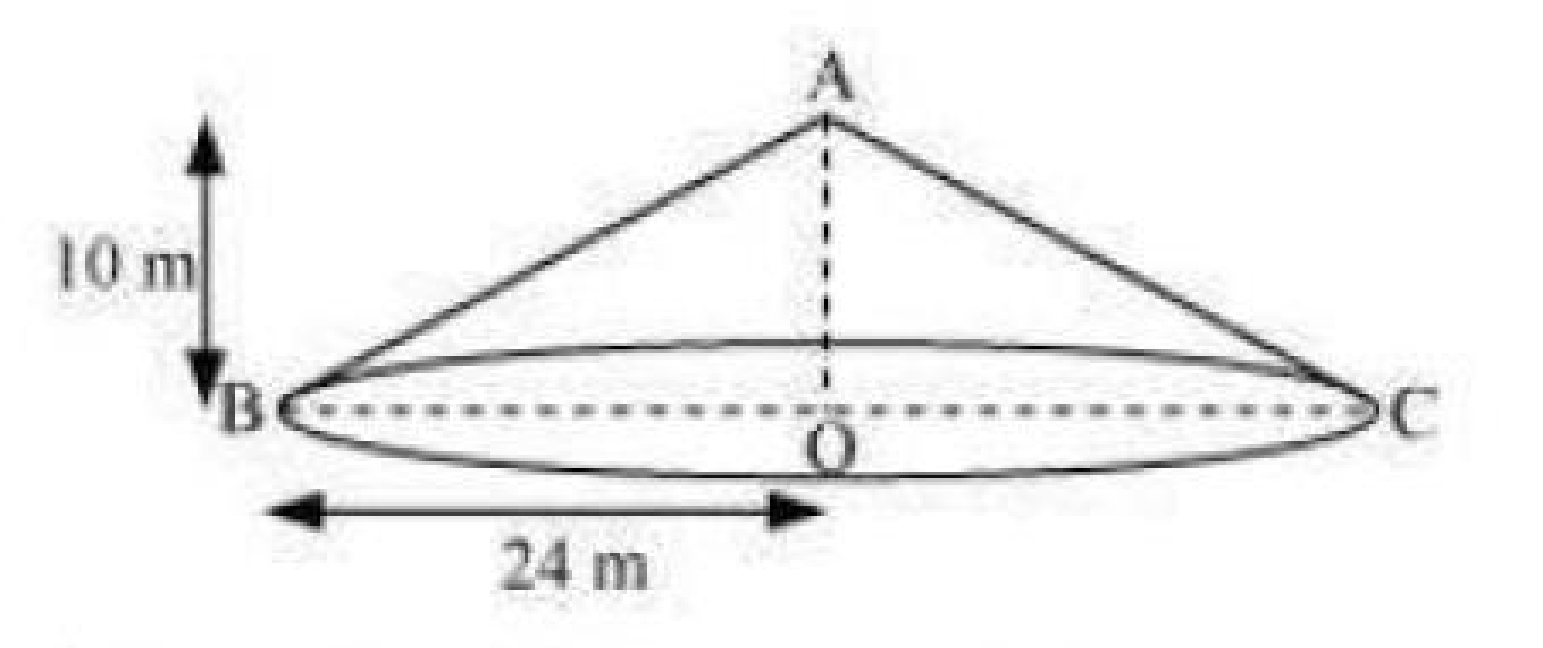# NCERT Solutions For Class 9 Math Chapter – 13 Exercise – 13.3

NCERT Solutions For Class 9 Math Chapter – 13 Exercise – 13.3

Q1. Diameter of the base of a cone is 10.5 cm and its slant height is 10 cm Find its curved surface area.

Solution:-

D = 10.5 cm

R = 10.5/2

L = 10 cm

Curved surface area = πrl

= 22/7 x 10.5/2 x 10

= 165 cm2.

Q2. Find the total surface area of a cone If its slant height is 21 m and diameter of its base is 24 m.

Solution:-

D = 24 m

R = 24/2 m = 12 m

L = 21 m

TSA of the cone = πr(l+r)

= 22/7x12mx(12m + 21m)

= 8712/7 m2

= 1244.57 m2

Q3.

Curved surface area of the cone is 308 m2 and its slant height is 14 cm Find

(ii) TSA of the cone.

Solution:-

R = r cm

L = 14 cm

Curved surface area = 308 cm2.

Πrl = 308

r = 308 cm2/πl

r = 308 cm2/14 cm x 7/22

r = 7 cm

Total Surface Area = π(l+r)

= 22/7 x7 x (7 + 14)

= 22 cm x 21 cm

= 462 cm2.

Q4. A conical tent is 10 m high and the radius of its base is 24 m Find

(i) Slant height of the tent.

(ii) Cost of the canvas required to make the tent if the costs of  1 m2 canvas is Rs 70.Solution:-

R = 24 m

H = 10 m

L = √r2 + h2

L = √(24 m)2 + (10 m)2

L = √576 m2 + 100 m2

L = √676 m2

L = 26 m

CSA of the cone = πrl

22/7 x 24 x 26

= 13728/7 m2

The cost of canvas required to make the tent at Rs 70 per m2 = 70 x 13728/7

= Rs 137280

Q5. What length of tarpaulin 3 m wide will be required to make conical tent of height 8 m and base radius 6 m ? Assume that the extra length of material that will be required for stitching margins and wastage in cutting is approximately 20 cm.

Solution:-

R = 6 m

H = 8 m

L = √(6)2 + (8)2

= 10 m

Curved Surface Area = πrl

= 3.14 x 6 x 10

= 188.4 m2

Width of the tarpaulin is 3 m

Area of the tarpaulin = 188.4 m2

Length of the tarpaulin = 188.4 / 3 m2 = 62.8 m

Extra length of the material = 20 cm = 20/100 = 0.2  m

Actual length required = 62.8 m + 0.2 m

= 63 m

Q6. The slant height and base diameter of a conical tomb are 25 m and 14 m respectively. Find the cost of white washing its curved surface at the rate of Rs 210 per 100 m2.

Solution:-

D = 14 m

R = 14/2 = 7 m

L = 25 m

CSA =  πrl

= 22/7 x 7 x 25

= 550 m2

Cost of the white washing at Rs 210 per 100 m2 = 210/100 x 550 = 1155

Q7. A joker cap is in the form of a right circular cone base radius 7 cm and height 24 cm Find the area of the sheet required to make 10 such caps.

Solution:-

R = 7 cm

H = 24 cm

L = √(7)2 + (24)2

L = √625 cm2

L = 25 cm

Area of the sheet required to make each cap = πrl

= 22/7 x 7 x 25

= 550 cm2.

Area of the sheet required to make 10 such caps = 10 x 550 cm2 = 5500 cm2

Q8. A bus stop is barricaded from the remaining part of the road by using 50 hollow cones made of recycled cardboard. Each cone has a base diameter of 40 cm and height 1 m. If the outer side of each of the cones is to be painted and the cost of painting is Rs 12 per m2 What wil be the cost of painting all these cones

Solution:-

D = 40 cm = 40/100 m = 0.04 m

R = 0.4/2 = 0.2 m

H = 1 m

L = √(0.2m)2 + (1m)2

= √0.04 m2 + 1 m‑2

= 1.02 m2

CSA = πrl

= 3.14 x 0.2 m x 1.02 m

= 0.64056 m2

CSA of 50 cones = 50 x 0.64056

= 32.028 m2

Cost of painting of 50 cones at Rs 12 per m2 = 32.028 x 12

= Rs 384.34